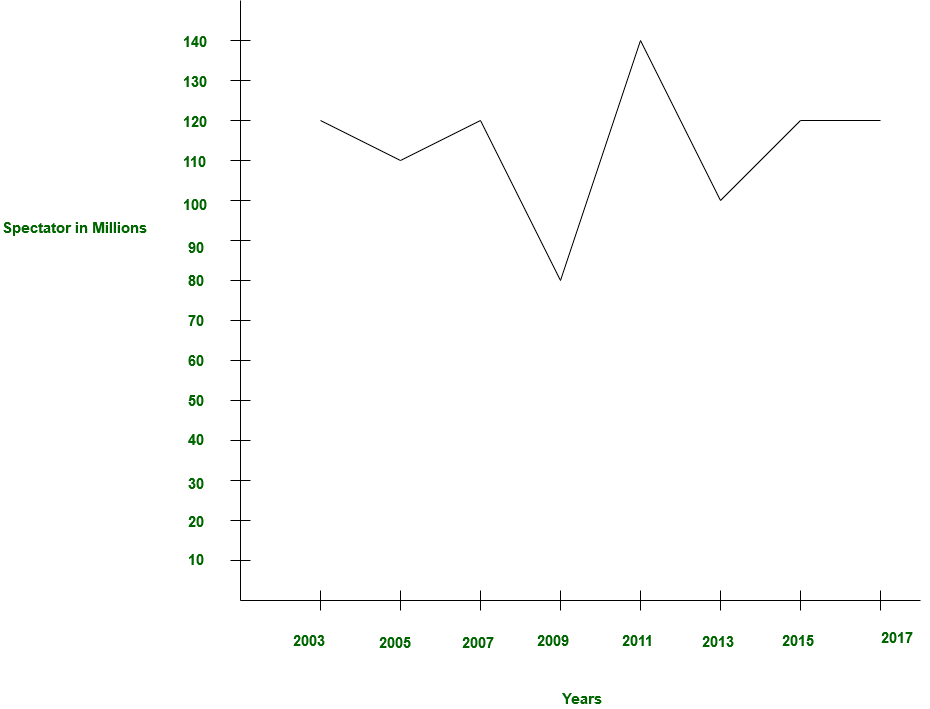Related Articles
GRE Quiz | GRE Quantitative 2 | Question 20
• Last Updated : 14 Mar, 2019

Question 18 – 20 are based on common data.

The histogram given below is showing the statistics for spectator in ICC tournament:In how many years the average the average number of spectator is equal to spectator in 2005?

(A) 2
(B) 3
(C) 4
(D) 5
(E) 6

Explanation: The number of spectator in 2005: 1,10,000 million,
In 2003 it was 1,20,000 million and in 2013 it was 1,00,000 million
In average,

```= (1,20,000 million + 1,00,000 million) / 2
= 1,10,000 million

Similarly 2013 and 2007

Similarly 2013 and 2015

Similarly 2013 and 2017

Similarly 2011 and 2009 ```

So, option (A) is correct.

Quiz of this Question

My Personal Notes arrow_drop_up